# ComplexMath

Library of mathematical functions as input/output blocks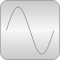# Package Contents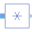Conj Output is equal to the conjugate complex input signal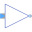Gain Output the product of a gain value with the input signal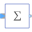Sum Output the sum of the elements of the input vector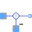Feedback Output difference between commanded input 1 and feedback input 2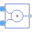Add Output the sum of the two inputs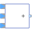Add3 Output the sum of the three inputs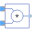Product Output product of the two inputs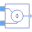Division Output first input divided by second input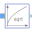Sqrt Output the square root of the input (= principal square root of complex number)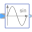Sin Output the sine of the input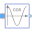Cos Output the cosine of the input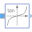Tan Output the tangent of the input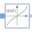Asin Output the arc sine of the input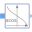Acos Output the arc cosine of the input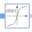Atan Output the arc tangent of the input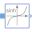Sinh Output the hyperbolic sine of the input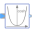Cosh Output the hyperbolic cosine of the input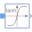Tanh Output the hyperbolic tangent of the input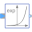Exp Output the exponential (base e) of the input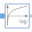Log Output the natural (base e) logarithm of the input (input <> '0' required)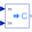RealToComplex Converts Cartesian representation to complex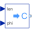PolarToComplex Converts polar representation to complex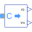ComplexToReal Converts complex to Cartesian representation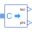ComplexToPolar Converts complex to polar representation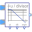Bode Calculate quantities to plot Bode diagram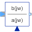TransferFunction Complex Transfer Function

# Information

This information is part of the Modelica Standard Library maintained by the Modelica Association.

This package contains basic mathematical operations, such as summation and multiplication, and basic mathematical functions, such as sqrt and sin, as input/output blocks. All blocks of this library can be either connected with continuous blocks or with sampled-data blocks.